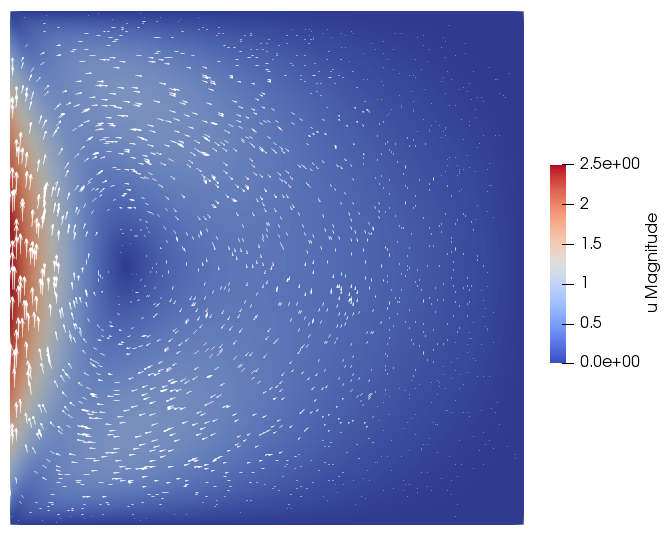# Navier-Stokes equation

We consider the incompressible Navier-Stokes equation in a domain $\Omega=(0,L_x)\times(0,L_y)$,

$\varrho(\partial_t\mathbf{u} + (\mathbf{u}\cdot\nabla)\mathbf{u}) - \nabla\cdot\big(2\nu\mathbf{D}(\mathbf{u})\big) - \nabla p = \mathbf{f},\qquad\text{ in }\Omega \\ \nabla\cdot\mathbf{u} = 0$

with velocity $\mathbf{u}$, pressure $p$, density $\varrho$, dynamic viscosity $\nu$, symmetric rate-of-strain tensor $\mathbf{D}(\mathbf{u})=\frac{1}{2}(\nabla\mathbf{u}+\nabla\mathbf{u}^\top)$, and external volume force $\mathbf{f}$, w.r.t. to boundary conditions $\mathbf{u}=\mathbf{g}$ on $\partial\Omega$. For an overview, see also Wikipedia.

## Discretization

### Weak formulation

In a weak variational formulation, we try to find $\mathbf{u}(t,\cdot)\in \mathbf{V}_\mathbf{g}:=\{\mathbf{v}\in H^1(\Omega)^d\,:\, \operatorname{tr}_{\partial\Omega}\mathbf{v} = \mathbf{g}\}$ and $p(t,\cdot)\in Q:=L_0^2(\Omega)$, such that

$\int_\Omega \varrho(\partial_t\mathbf{u} + (\mathbf{u}\cdot\nabla)\mathbf{u})\cdot\mathbf{v} + \nu\nabla\mathbf{u}:\nabla\mathbf{v} + p\,\nabla\cdot\mathbf{v}\,\text{d}\mathbf{x} = \int_\Omega \mathbf{f}\cdot\mathbf{v}\,\text{d}\mathbf{x},\qquad\forall \mathbf{v}\in \mathbf{V}_0, \\ \int_\Omega q\,\nabla\cdot\mathbf{u}\,\text{d}\mathbf{x} = 0,\qquad \forall q\in Q.$

for $t\in[0,T]$, given $\mathbf{u}(0,\cdot)\equiv\mathbf{u}^0$.

The pair $\big(\mathbf{u}(t,\cdot), p(t,\cdot)\big)$ is thus in the product space $\mathbf{V}_\mathbf{g}\times Q$ where $\mathbf{V}_\mathbf{g}$ is also a product of $H^1$ spaces, i.e., $\mathbf{V}_\mathbf{g}=V_{g_0}\times V_{g_1}\times V_{g_2}\simeq [V_{g_i}]^d$. Later, this space will be approximated with a Taylor-Hood space of piecewise quadratic and linear functions.

### Time-discretization

A linearized time-discretization with a semi-implicit backward Euler scheme then reads:

For $k=0,1,2,\ldots, N$ find $\mathbf{u}^{k+1}\in\mathbf{V}_\mathbf{g},\, p\in Q$, s.t.

$\int_\Omega \varrho\Big(\frac{1}{\tau}(\mathbf{u}^{k+1} - \mathbf{u}^k) + (\mathbf{u}^k\cdot\nabla)\mathbf{u}^{k+1}\Big)\cdot\mathbf{v} + \nu\nabla\mathbf{u}^{k+1}:\nabla\mathbf{v} + p\,\nabla\cdot\mathbf{v}\,\text{d}\mathbf{x} \\ = \int_\Omega \mathbf{f}(t^{k+1})\cdot\mathbf{v}\,\text{d}\mathbf{x},\qquad\forall \mathbf{v}\in \mathbf{V}_0, \\ \int_\Omega q\,\nabla\cdot\mathbf{u}^{k+1}\,\text{d}\mathbf{x} = 0,\qquad \forall q\in Q.$

with $0=t^0, timestep $\tau=t^{k+1} - t^k$, and given initial velocity $\mathbf{u}^0$.

### Finite-Element formulation

Let $\Omega$ be partitioned into quasi-uniform elements by a conforming triangulation $\mathcal{T}_h$. The functions $\mathbf{u}$ and $p$ are approximated by functions from the discrete functionspace $\mathbb{T}_h := [\mathbb{V}_{2,\,g_i}]^d\times \mathbb{V}_1$ with $\mathbb{V}_{p} := \{v\in C^0(\Omega)\,:\, v|_T\in\mathbb{P}_p(T),\, \forall T\in\mathcal{T}_h\}$ and $\mathbb{V}_{p,\,g} := \{v\in \mathbb{V}_p\,:\,\operatorname{tr}_{\partial\Omega} v=g\}$, where $\mathbb{P}_p$ is the space of polynomials of order at most $p$. The space $\mathbb{T}_h$ will be denoted as Taylor-Hood space.

Let Grid grid be the Dune grid type representing the triangulation $\mathcal{T}_h$ of the domain $\Omega$, then the basis of the Taylor-Hood space can be written as a composition of Lagrange bases:

using namespace Dune::Functions::BasisFactory;
auto basis = composite(
power<Grid::dimensionworld>(
lagrange<2>(), flatInterleaved()),
lagrange<1>(), flatLexicographic());

At this point, any Dirichlet boundary conditions are ignored in the definition of the bases. We build the Taylor-Hood basis as a composition of a product of $d\times\mathbb{V}_2$ bases for the velocity components and a $\mathbb{V}_1$ basis for the pressure. We have to use composite(...) to combine the different types for velocity and pressure space, while we can use power<d>(...) for the d velocity component spaces of the same type.

For efficiency, we use flat indexing strategies flatInterleaved and flatLexicographic to build a global continuous numbering of the basis functions.

See the tutorial Grids and Discrete Functions for more details.

## Implementation

We split the implementation into three parts, 1. the time derivative, 2. the stokes operator and 3. any external forces. For addressing the different components of the Taylor-Hood basis, we introduce the treepaths _v = Dune::Indices::_0 and _p = Dune::Indices::_1 for velocity and pressure, respectively.

### Problem framework

We have an in-stationary problem consisting of a sequence of stationary equations for each timestep, thus we combine a ProblemInstat with a ProblemStat .

using namespace AMDiS;
ProblemStat prob{"stokes", grid, basis};
prob.initialize(INIT_ALL);

ProblemInstat probInstat{"stokes", prob};
probInstat.initialize(INIT_UH_OLD);

For convenience, we use class template argument deduction here.

### Time derivative

For simplicity of this example, we use a backward Euler time stepping and a linearization of the nonlinear advection term:

// define a constant fluid density
double density = 1.0;
Parameters::get("stokes->density", density);

// a reference to 1/tau
auto invTau = probInstat.invTau();

// <1/tau * u, v>
auto opTime = makeOperator(tag::testvec_trialvec{},
density * invTau);
prob.addMatrixOperator(opTime, _v, _v);

// <1/tau * u^old, v>
auto opTimeOld = makeOperator(tag::testvec{},
density * invTau * probInstat.oldSolution(_v));
prob.addVectorOperator(opTimeOld, _v);

for (int i = 0; i < Grid::dimensionworld; ++i) {
// <(u^old * nabla)u_i, v_i>
auto opNonlin = makeOperator(tag::test_gradtrial{},
density * prob.solution(_v));
prob.addMatrixOperator(opNonlin, makeTreePath(_v,i), makeTreePath(_v,i));
}


### Stokes operator

The stokes part of the Navier-Stokes equation is a common pattern that emerges in several fluid equations.

$\int_\Omega \nu\nabla\mathbf{u}:\nabla\mathbf{v} + p\,\nabla\cdot\mathbf{v} + q\,\nabla\cdot\mathbf{u}\,\text{d}\mathbf{x}$

for $\mathbf{u},\mathbf{v}\in V$ and $p,q\in Q$.

// define a constant fluid viscosity
double viscosity = 1.0;
Parameters::get("stokes->viscosity", viscosity);

for (int i = 0; i < Grid::dimensionworld; ++i) {
// <viscosity*grad(u_i), grad(v_i)>
auto opL = makeOperator(tag::gradtest_gradtrial{}, viscosity);
prob.addMatrixOperator(opL, makeTreePath(_v,i), makeTreePath(_v,i));
}

// <d_i(v_i), p>
auto opP = makeOperator(tag::divtestvec_trial{}, 1.0);
prob.addMatrixOperator(opP, _v, _p);

// <q, d_i(u_i)>
auto opDiv = makeOperator(tag::test_divtrialvec{}, 1.0);
prob.addMatrixOperator(opDiv, _p, _v);

where we have used the identity

$\nabla\mathbf{u}:\nabla\mathbf{v} = \sum_i \nabla u_i\cdot\nabla v_i$

### External volume force

The force may be implemented as a vector-valued function of the global coordinates or any other expression that leads to a local force vector at the quadrature points, e.g.

using WorldVector = typename Grid::template Codim<0>::Geometry::GlobalCoordinate;
auto opForce = makeOperator(tag::testvec{},
[](WorldVector const& x) { return WorldVector{1.0, 0.0}; });
prob.addVectorOperator(opForce, _v);


## Numerical example

We consider a lid-driven cavity problem in a square domain $\Omega$ with $L_x=L_y=1$ no external force, and with Dirichlet boundary conditions

$\mathbf{g}(\mathbf{x}) = \begin{pmatrix}0 \\ v_1 x_1(1 - x_1)(1 - x_0)\end{pmatrix}$

Thus, the boundary value is zero on top, right, and bottom boundary and parabolic on the left boundary. The boundary condition is implemented, by first setting boundary ids for parts of the grid boundary and second, setting the Dirichlet value:

double vel = 1.0;
Parameters::get("stokes->boundary velocity", v1);

// define boundary values
auto g = [vel](WorldVector const& x)
{
return WorldVector{0.0, v1*x*(1.0 - x)*(1.0 - x)};
};

// set boundary conditions for velocity
prob.boundaryManager()->setBoxBoundary({1,1,1,1});
prob.addDirichletBC(1, _v, _v, g);


### Simulation

The time-stepping process with a stationary problem in each iteration can be started using an AdaptInstationary manager class:

// set initial conditions
prob.solution(_v).interpolate(g);

// start simulation
AdaptInfo adaptInfo("adapt");
AdaptInstationary adapt("adapt", prob, adaptInfo, probInstat, adaptInfo);
adapt.adapt();


## Complete Example

The full source code of the Navier-Stokes example can be found in the repository at examples/navier_stokes.cc.

Compile with

cmake --build build --target navier_stokes.2d


and run with

./build-cmake/examples/navier_stokes.2d examples/init/navier_stokes.dat.2d


The flow field with density=1, viscosity=1 and boundary velocity=10 will look like